To celebrate special occasions like Father's Day, I like to relax with a cup of coffee and read the newspaper. When I looked at the weather page, I was astonished by the seeming uniformity of temperatures across the contiguous US. The weather map in my newspaper was almost entirely yellow and orange, which told me that the midday temperatures were predicted to have extremely small variation. It looked like the range of temperatures might vary by less than 30 degrees Fahrenheit (17 degrees Celsius), with most temperatures in the 70's and 80's.

To me this seemed like an extremely small variation, so that afternoon I downloaded the actual midday temperatures from Weather.com, which are shown below: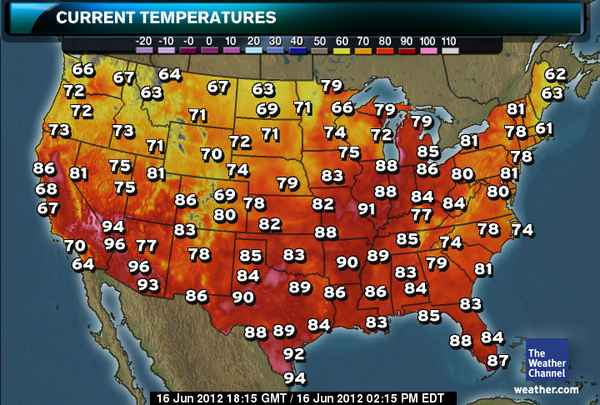The Weather.com map uses a different color scale than my local paper. Nevertheless I quickly estimated that most of the temperatures were between 62 F and 92 F, with just a few hot spots in southern Texas, Arizona, and Nevada. The statistician in me wondered, what is the record for the "most uniform" temperatures at midday in the contiguous US? I'll bet that someone can automate the downloading of temperatures for standard US locations and answer the question. I'd love to see a graph of "temperature variation vs. date" for, say, the last ten or twenty years.

I don't know of a source for temperature data, but I figured that I might as well do SOME statistics to celebrate Father's Day weekend! I enlisted my youngest child to assist me in typing in the temperatures. (Yes, it was a father/daughter bonding moment.) I ran the following univariate analysis of the temperatures:

```data Temps06162012; input Temperature @@; datalines; 66 72 72 73 86 81 68 67 70 64 64 67 63 73 71 75 81 75 94 96 77 96 93 67 71 72 70 74 69 86 80 83 78 86 63 69 71 71 79 78 82 83 85 84 89 90 88 89 92 94 79 66 79 74 72 75 88 83 88 82 91 88 89 90 86 86 83 84 79 81 85 86 80 84 84 77 85 74 79 83 84 85 62 63 81 61 78 78 81 80 74 78 81 83 84 88 87 ;   proc univariate data=Temps06162012; var Temperature; histogram Temperature; run;```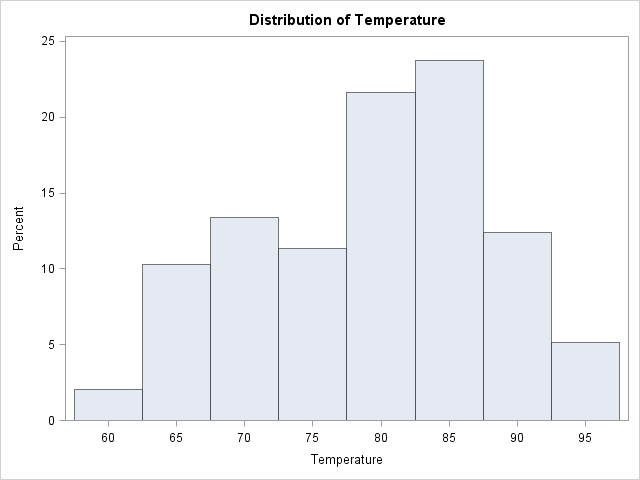The histogram and the accompanying tabular output told me that the mean temperature was a comfortable 79 degrees F, and that the standard deviations of temperatures was a paltry 8.56 degrees F. It was, indeed, a beautiful weekend for celebrating Father's Day.

Can anyone find a date for which the temperatures exhibited less variation? I'd love to see the analysis.The other day I encountered an article in the SAS Knowledge Base that shows how to write a macro that "returns the variable name that contains the maximum or minimum value across an observation." Some people might say that the macro is "clever." I say it is complicated. This is a simple problem; it deserves a simple solution.

This is one of those situations where a SAS/IML implementation is simpler and cleaner than a macro/DATA step solution. The following DATA step creates the data that are used in the SAS Knowledge Base article:

```/* Data for Sample 46471: Return the variable name that contains the max or min value across an observation */ data one; input a b c d e; cards; 1 3 12 6 15 34 583 294 493 2 ; run;```

By inspection, the minimum value in the first row is 1, which occurs for the variable A. In the second row, the minimum value is 2, which occurs for the variable E.

To find the variable for each row that contains the minimum value for that row, you can use the index minimum subscript reduction operator, which has the symbol >:<. The subscript reduction operators are a little-known part of the SAS/IML language, but they can be very useful. The following SAS/IML program begins by reading all numerical variables into a matrix, X. The subscript reduction operator then computes a column vector whose ith element is the column for which the ith row of X is minimal. You can use this column vector as an index into the names of the columns of X.

```/* For each row, find the variable name corresponding to the minimum value */ proc iml; use one; read all var _NUM_ into X[colname=VarNames]; close one;   idxMin = X[, >:<]; /* find columns for min of each row */ varMin = varNames[idxMin]; /* corresponding var names */ print idxMin varMin;```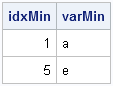Yes, those two statements compute the same quantity as the complicated macro. And, if you are willing to nest the statements, you can combine them into a single statement:
varNames[X[, >:<]].

Finding the maximum value for each row is no more difficult: simply use the <:> subscript reduction operator.

The macro featured in the Knowledge Base article includes an option to compute with specified variables, rather than all numerical variables. That, too, is easily accomplished. For example, the following statements find the variable that has the largest value among the A, B, and C variables:

```varNames = {a b c}; use one; read all var varNames into X; close one;   idxMax = X[,<:>]; varMax = varNames[idxMax]; print idxMax varMax;```My next post will discuss subscript reduction operators in further details.

To my readers who are SQL experts: Is there a simple way to solve this problem by using PROC SQL? Leave a comment.I want to create a vector as follows. Suppose there are two given vectors x=[A B C] and f=[1 2 3]. Here f indicates the frequency vector. I hope to generate a vector c=[A B B C C C]. I am trying to use the REPEAT function in the SAS/IML, language but there is always something wrong. Can you help me?

This is probably a good time to remind everyone about the SAS/IML Community (formerly known as a Discussion Forum). You can post your SAS/IML questions there 24 hours a day. That is always a better plan than making a personal appeal to me, because I receive dozens of questions like this every month, and there is no way that I can personally reply. There are lots of experienced SAS/IML experts out there, so please use the SAS/IML Community to tap into that knowledge.

That said, I think the answer to this reader's question makes an interesting example of statistical programming with SAS/IML software. It is trivial to solve this in the DATA step (see the end of this article), but how might you solve it in the SAS/IML language? If you'd like to try to solve this problem yourself, stop reading here. Spoilers ahead!

### Create a new vector that duplicates frequencies

The goal is to write a function that duplicates or "expands" data that have a frequency variable. The important function to use for this task is the CUSUM function, which computes the cumulative frequencies. Let's look at a simple example and apply the CUSUM function to the frequency vector:

```proc iml; values={A,B,C,E}; freq = {2,1,3,4}; cumfreq = cusum(freq); print values freq cumfreq;```As shown in the output, the cumfreq variable contains the indices for the expanded data. The expanded data will be a vector that contains 10 elements. The first data value (A) repeats twice (the freq value), so it repeats until element 2 (the cumfreq value) in the expanded vector. The second category fills element 3. The next category repeats 3 times, so it occupies up through element 6 in the expanded vector. The last category repeats until element 10. The following DO loop specifies each data value and the indices of the expanded vector that it should occupy:

```print (values)[label="value"] (1:cumFreq)[label="Indices"]; do i = 2 to nrow(values); bIdx = 1 + cumFreq[i-1]; /* begin index */ eIdx = cumFreq[i]; /* end index */ value = values[i]; print value (bIdx:eIdx)[label="Indices"]; end;```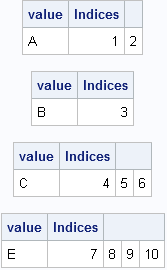The output shows that we have all the information we need to allocate a vector of length 10 and fill it with the data values, where the ith value is repeated freq[i] times. The key, it turns out, is to use the CUSUM function to find the indices that correspond to the each data value.

### A module to compute the expanded data

In SAS procedures that support a FREQ statement, the frequency values must be positive integers. If the frequency value is missing or is a nonpositive value, the corresponding data value is excluded from the analysis. It is easy to add that same feature to a module that takes a vector of values and a vector of frequencies and returns a vector that contains the data in expanded form. This is implemented in the following SAS/IML module, which allocates the result vector with the first data value in order to avoid handling the first element outside of the DO loop:

```start expandFreq(_x, _freq); /* Optional: handle nonpositive and fractional frequencies */ idx = loc(_freq > 0); /* trick: in SAS this also handles missing alues */ if ncol(idx)=0 then return (.); x = _x[idx]; freq = round( _freq[idx] );   /* all frequencies are now positive integers */ cumfreq = cusum(freq);   /* Initialize result with x to get correct char/num type */ N = nrow(x); expand = j(cumfreq[N], 1, x); /* useful trick */   do i = 2 to N; bIdx = 1 + cumFreq[i-1]; /* begin index */ eIdx = cumFreq[i]; /* end index */ expand[bIdx:eIdx] = x[i];/* you could use the REPEAT function here */ end; return ( expand ); finish;   /* test the module */ values={A,B,C,D,E,F}; freq = {2,1,3,0,4,.}; /* include nonpositive and missing frequencies */ y = expandFreq(values, freq); print values freq y;```Notice that you don't actually need to use the REPEAT function because SAS/IML is happy to assign a scalar value into a vector. The scalar is automatically repeated as often as needed in order to fill the vector.

### A DATA step solution

As indicated at the beginning of this post, the DATA step solution is quite simple: merely use the OUTPUT statement in a loop, as shown in the following example:

```data Orig; input x \$ Freq; datalines; A 2 B 1 C 3 D 0 E 4 F . ; run;   /* expand original data by frequency variable */ data Expand; keep x; set Orig; if Freq<1 then delete; do i = 1 to int(Freq); output; end; run; proc print data=Expand; run;```

The output data set contains the same data as the y vector in the SAS/IML program.SAS software provides many run-time functions that you can call from your SAS/IML or DATA step programs. The SAS/IML language has several hundred built-in statistical functions, and Base SAS software contains hundreds more. However, it is common for statistical programmers to extend the run-time library to include special user-defined functions.

In a previous blog post I discussed two different ways to apply a log transformation when your data might contain missing values and negative values. I'll use the log transformation example to show how to define and call user-defined functions in SAS/IML software and in Base SAS software.

### A "safe" log transformation in the SAS/IML language

In the SAS/IML language, it is easy to write user-defined functions (called modules) that extend the functionality of the language. If you need a function that safely takes the natural logarithm and handles missing and negative values, you can easily use the ideas from my previous blog post to create the following SAS/IML function:

```proc iml; /* if Y>0, return the natural log of Y otherwise return a missing value */ start SafeLog(Y); logY = j(nrow(Y),ncol(Y),.); /* allocate missing */ idx = loc(Y > 0); /* find indices where Y > 0 */ if ncol(idx) > 0 then logY[idx] = log(Y[idx]); return(logY); finish;   Y = {-3,1,2,.,5,10,100}; LogY = SafeLog(Y); print Y LogY;```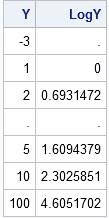The program is explained in my previous post, but essentially it allocates a vector of missing values and then computes the logarithm for the positive data values. The START and FINISH statements are used to define the SafeLog function, which you can then call on a vector or matrix of values.

In this example, the function is defined only for the current PROC IML session. However, you can store the function and load it later if you want to reuse it.

### Defining a "safe" log transformation by using PROC FCMP

You can also extend the Base SAS library of run-time functions. The FCMP procedure enables you to define your own functions that can be called from the DATA step and from other SAS procedures. (The MCMC procedure has an example of calling a user-defined function from a SAS/STAT procedure.) If you have never used the FCMP procedure before, I recommend Peter Eberhardt's 2009 paper on defining functions in PROC FCMP. For a more comprehensive treatment, see Jason Secosky's 2007 paper.

Technically, you don't need to do anything special in the DATA step if you want a SAS missing value to represent the logarithm of a negative number: the DATA step does this automatically. However, the DATA step also generates some scary-looking notes in the SAS LOG:

```NOTE: Invalid argument to function LOG at line 72 column 5.
RULE:      ----+----1----+----2----+----3----+----4----+----5----+----6----+----7----+----8----+
74         -3 1 2 . 5 10 100
x=-3 y=. _ERROR_=1 _N_=1
NOTE: Missing values were generated as a result of performing an operation on missing values.
NOTE: Mathematical operations could not be performed at the following places. The results of
the operations have been set to missing values.
```

I prefer my programs to run with a clean, healthy-looking SAS LOG, so I will use PROC FCMP to define a SafeLog function that has the same behavior (and name!) as my SAS/IML function:

```proc fcmp outlib=work.funcs.MathFuncs; function SafeLog(y); if y>0 then return( log(y) ); return( . ); endsub; quit;```

The function returns a missing value for nonpositive and missing values. The definition of the function is stored in a data set named WORK.FUNCS, which will vanish when you exit SAS. However, you can create the definition in a permanent location if you want to call the function in a later SAS session.

In order to call the function from the DATA step, use the CMPLIB= option, as shown in the following example:

```options cmplib=work.funcs; /* define location of SafeLog function */ data A; input x @@; y = SafeLog(x); /* test the function */ datalines; -3 1 2 . 5 10 100 ; run;```

The result is not shown, but it is identical to the output from the SAS/IML program.

You might not have need for the SafeLog function, but it is very useful to know how to define user-defined functions in SAS/IML software and in Base SAS software. SAS/IML modules and PROC FCMP functions make it easy to extend the built-in functionality of SAS software.Because the SAS/IML language is a general purpose programming language, it doesn't have a BY statement like most other SAS procedures (such as PROC REG). However, there are several ways to loop over categorical variables and perform an analysis on the observations in each category.

One way is to use the powerful UNIQUE-LOC technique, which I have blogged about several times and wrote about in my book, Statistical Programming with SAS/IML Software. This is a convenient technique when all of the data fit into memory and when there is a single BY variable.

However, sometimes you might need to process data that does not fit into RAM. In this case, you can use a DO loop to iterate over the categories and use WHERE processing inside the loop to read only a single BY group into memory (assuming each BY group fits). Furthermore, you might want to analyze the joint levels of several categorical variables, and it easy to compute the joint levels by using PROC FREQ.

### Computing the BY groups

Suppose that you want to analyze the observations that correspond to joint categories of the Sashelp.Cars data set for the ORIGIN and TYPE variables. You can find the joint categories (and the number of observations in each) by using PROC FREQ, as shown in the following example:

```/* find unique BY-group combinations */ proc freq data=Sashelp.Cars; tables Origin*Type / out=FreqOut; run; proc print data=FreqOut; run;```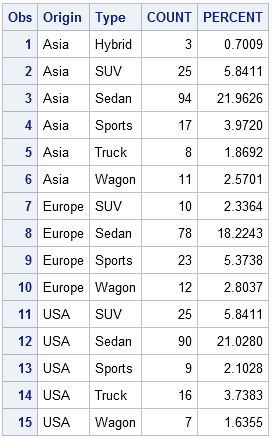Notice that some potential 3x6 combinations do not appear in the data. For example, these data do not contain a European or American hybrid vehicle, nor a European truck.

Now suppose that you want to iterate over these 15 categories and do an analysis of the observations that belong to each. The next section shows how to proceed if the data set is so large that it doesn't fit into memory, but each individual BY group does fit into memory.

### Analyzing BY groups in PROC IML

For the sake of the example, I'll compute a trivial statistic: the mean of each BY group. Doing this computation in SAS/IML software is completely unnecessary because you can compute it more efficiently with PROC MEANS, but the point of this example is to show how you can access the observations in each BY group within the SAS/IML language.

The following program reads the joint categories (the BY group levels) from the FreqOut data set into SAS/IML vectors. Then, for each BY group, it uses a READ statement with a WHERE clause to read only the observations in that BY group.

```proc iml; /* read unique BY groups */ use FreqOut nobs NumObs; read all var {origin type}; close FreqOut;   varNames = {"MPG_City" "MPG_Highway"}; /* analysis variables */ use Sashelp.Cars; /* open data set for reading */ Mean = j(NumObs, ncol(varNames)); /* allocate results */ do i = 1 to NumObs; /* for each BY group */ read all var varNames into X /* read data for the i_th group */ where(Origin=(origin[i]) & Type=(type[i])); /* X contains data for i_th group; analyze it */ Mean[i,] = mean(X); end; close Sashelp.Class;   print Origin Type Mean[label="" colname=varNames format=4.1];```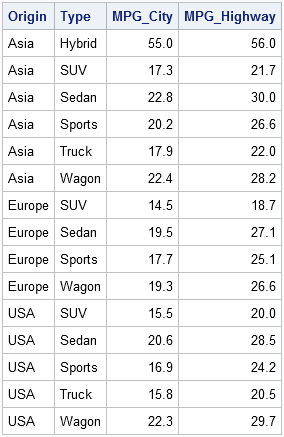A few comments on this program:

• When the FreqOut data set is read, the NOBS keyword is used to store the number of observations (15) into the NumObs variable. This value is used to allocate the Mean array and to control the number of iterations in the DO loop.
• The program is not efficient in terms of reading the data. Notice that the program passes through the data 15 times, once for each BY group.
• Notice the syntax of the WHERE clause. In the expression WHERE(Origin=(origin[i])), the left side of the equal sign specifies a variable in the data set. The expression on the right side of the equal sign returns the ith element of the origin vector, which was read from the FreqOut data set. The parentheses around the expression are required.

I'll repeat this isn't an efficient way to read data compared to reading it all at once. However, this technique might be necessary when the data set is large. It can also be used when the groups that should be analyzed are specified in a data set such as FreqOut. It is not necessary that the data set contain all levels of the BY groups. For example, if you want to analyze data for a handful of vehicle types (or states, or counties, ...), you can use this approach to read only the data for groups that you are interested in.

This same technique is also a way to avoid calling a SAS/IML program in a macro %DO loop. Some programmers like to write a program that handles a single subgroup and then use a macro loop to subset their data and call the program on each subset. (For example, the program might analyze one year's worth of data, and you want to call it for all the years 1990–2010.) I find it difficult to debug and modify programs that have lots of macro variables and are embedded in a macro loop. Therefore I prefer the technique presented here, which avoid using macro statements.Last week I discussed how to fit a Poisson distribution to data. The technique, which involves using the GENMOD procedure, produces a table of some goodness-of-fit statistics, but I find it useful to also produce a graph that indicates the goodness of fit.

For continuous distributions, the quantile-quantile (Q-Q) plot is a useful diagnostic plot for checking whether a model distribution fits the data. As I mentioned in a previous article, you can create a discrete Q-Q plot, but it suffers from overplotting and is not usually used for discrete distributions.

So what can you use instead? One option is to use the Poisonness plot introduced by Hoaglin and Tukey (1985) and shown in the following image. If a Poisson model fits the data, then the points on this plot will fall along a straight line, so the plot is similar in usage to the familiar Q-Q plot. The Poissonness plot is outlined by Martinez and Martinez (2002), Computational Statistics Handbook with MATLAB, and the SAS/IML program in this article is based on their MATLAB code.The Poissonness plot is also described by Michael Friendly in his excellent book, Visualizing Categorical Data, which is a "must have" book for any statsitician who models categorical data. Friendly provides a POISPLOT macro that creates the Poissonness plot. For other discrete distributions, Friendly provides the DISTPLOT macro, which provides diagnostic plots for fitting Poisson, Binomial, Negative Binomial, Geometric, or Log Series distributions to data.

### Preparing the data

To introduce the Poissonness plot, I'll use the same data as in my previous post: the number of emails that I received during half-hour periods for one weekday at work:

```/* number of emails received in each half-hour period 8:00am - 5:00pm on a weekday. */ data MyData; input N @@; datalines; 7 7 13 9 8 8 9 9 5 6 6 9 5 10 4 5 3 8 4 ; run;```

The question is whether the data can be described by a Poisson model. Equivalently, did I receive emails at a constant rate? To begin the analysis, it is useful to introduce zero counts for the categories (such as 11 and 12) that did not appear in the data. This can be accomplished by the following steps:

```/* create a data set that contains all categories of interest */ data AllCat / view=AllCat; do N = 0 to 13; output; end; run; /* merge all categories with the observed data */ data Table; set AllCat MyData(in=_w); w = _w; /* create weight variable: 0 or 1 */ run; /* tabulate counts */ proc freq data=Table; weight w / zeros; /* include obs with 0 weight */ tables N / out=FreqOut nocum; /* SPARSE option is on b/c of ZEROS option */ run;```### Creating a Poissonness plot

The simplest form of the Poissonness plot graphs the quantity φ(fk) = log(k! fk/F) against the category k, where fk is the observed frequency for category k and F is the total number of observations. For my data, k=0,1,...,13 and F=19. Hoaglin and Tukey also suggest using a different symbol for any category that has only one observation because φ has more variablitity for categories with a small number of counts.

The following SAS/IML prorgam does the following:

```/* algorithm from Martinez and Martinez (2002), p. 127 */ proc iml; /* if Y>0, return the natural log of Y, otherwise return a missing value http://blogs.sas.com/content/iml/2011/04/27/log-transformations-how-to-handle-negative-data-values/ */ start SafeLog(Y); logY = j(nrow(Y),1,.); /* allocate missing */ idx = loc(Y > 0); /* find indices where Y > 0 */ if ncol(idx) > 0 then logY[idx] = log(Y[idx]); return(logY); finish;   /* return the Hoaglin-Tukey (1985) phi function for a table of counts. */ /* args: output output input input */ start Poissonness(phi, SampleIsSmall, k, Freq); phi = SafeLog(fact(k) # Freq / sum(Freq) ); SampleIsSmall = choose(Freq=1, 1, 0); /* indicator variable, Freq=1 */ finish;   use FreqOut; read all var {N Count}; close FreqOut; run Poissonness(phi, SampleIsSmall, N, Count); create Poissonness var{"N" "Count" "Phi" "SampleIsSmall"}; append; close Poissonness;```

The following call to SGPLOT creates the Poissonness plot. Notice that I am changing the default ODS style (for SAS 9.3) because I want to classify the points according the value of the indicator variable SampleIsSmall. I want the markers that correspond to small samples to have a differnt color and a different symbol than the other markers. Therefore I use the HTMLBLUECML style, where the "CML" means that Colors, Markers, and Lines all change to reflect group membership.

```ods html style=htmlbluecml; /* SAS 9.3 */   proc sgplot data=Poissonness; scatter x=N y=Phi / group=SampleIsSmall markerattrs=(size=10pt); xaxis grid type=discrete; yaxis grid; title "Hoaglin-Tukey Poissonness Plot"; run;```

The plot is shown at the top of this article. Notice that the points on this graph fall near a straight line, which indicates that the Poisson distribution is a good model for these data.

### A Modified Poissonness plot

Hoaglin and Tukey (1985) suggest a small modfication to the φ function that adjusts the position of categories with small counts. If you prefer, use the following modified SAS/IML routine. The corresponding plot is only slightly different for these data and is not shown.

```/* return the modified Hoaglin-Tukey (1985) phi function for a table of counts. */ /* args: output output input input */ start Poissonness(phi, SampleIsSmall, k, Freq); Total = sum(Freq); pHat = Freq / Total; correction = Freq - 0.67 - 0.8*pHat; FreqStar = choose(Freq=0, ., choose(Freq=1, 1/constant("E"), correction)); phi = SafeLog(fact(k) # FreqStar / Total); SampleIsSmall = choose(Freq=1, 1, 0); /* indicator variable, Freq=1 */ finish;```Last week I blogged about how to construct a smoother for a time series for the temperature in Albany, NY from 1995 to March, 2012. I smoothed the data by "folding" the time series into a single "year" that contains repeated measurements for each day of the year.

Experts in time series analysis would use different techniques to analyze these data, so today I'm giving my colleague, Udo Sglavo, a chance to comment on how he might analyze these data. I am not knowledgeable about the Singular Spectrum Analysis method that he presents, but it seems like a powerful mixture of principal component analysis and orthogonal decomposition analysis in the time domain. Enjoy this guest post!

-- RickRick’s blog about smoothers for periodic data caught my attention because I recently read a SAS Global Forum paper, "An Introduction to Singular Spectrum Analysis with SAS/ETS Software" by M. Leonard, et al. Singular spectrum analysis is a relatively new approach to modeling long time series data for which patterns (such as trends and cycles) are difficult to visualize and analyze. The general idea of singular spectrum analysis is to apply nonparametric techniques for decomposing time series data into principal components. Since the paper also used temperature data as an example, I thought it would be fun to apply singular spectrum analysis to the Albany temperature data.

It is good practice to visualize the data first, so I plotted the complete time series and the seasonal cycles using monthly temperature averages. You can plot the data by using the TIMESERIES procedure in SAS/ETS software:

```proc timeseries data=tempdata out=_null_ plot=(series cycles); title "Average Temperature per Month"; id date interval=month accumulate=average; var temperature; run;```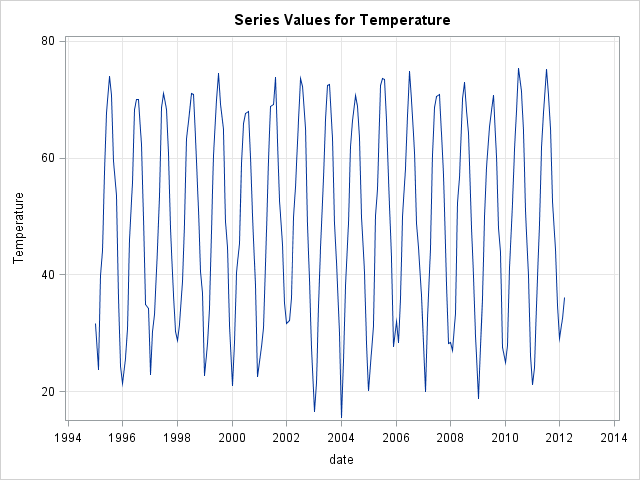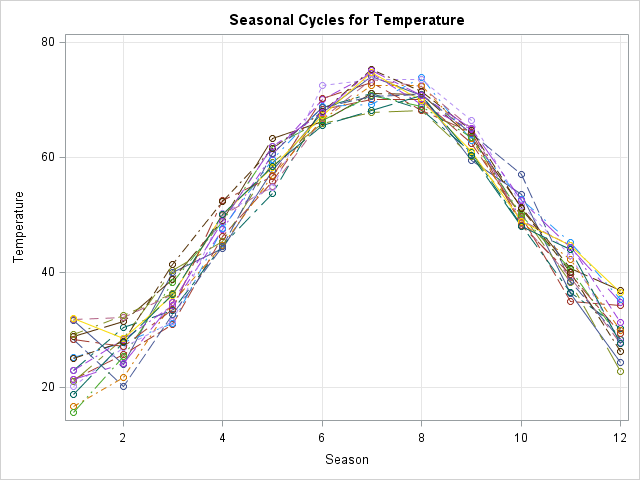As expected the temperature data shows a strong seasonal behavior with high average temperatures in summer and low average temperatures in winter.

As a next step, I applied a singular spectrum analysis to decompose the data into two main components:

```proc timeseries data=tempdata out=_null_ plot=SSA; title "SSA Analysis: Average Temperature per Month"; id date interval=month accumulate=average; SSA / LENGTH=12 GROUPS=(1)(2 3); var temperature; run;```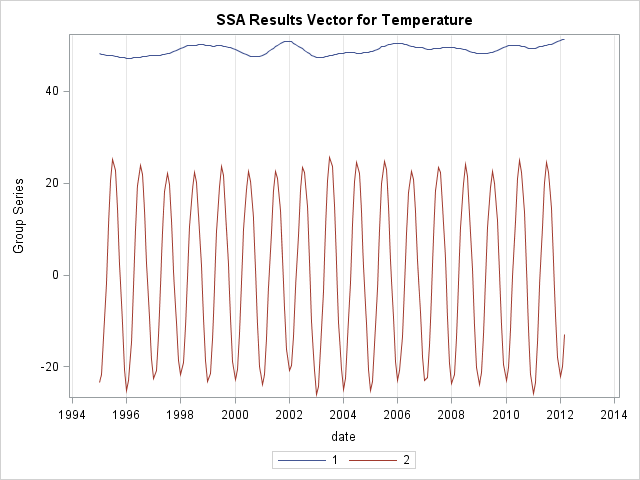The first component (labeled 1) seems to reflect the long-term trend, whereas the second component (labeled 2) reflects the cyclic behavior of the temperatures. When overlaying individual components with the original series, this relationship becomes even more apparent. For example, the following plot shows that the first component looks like a moving average: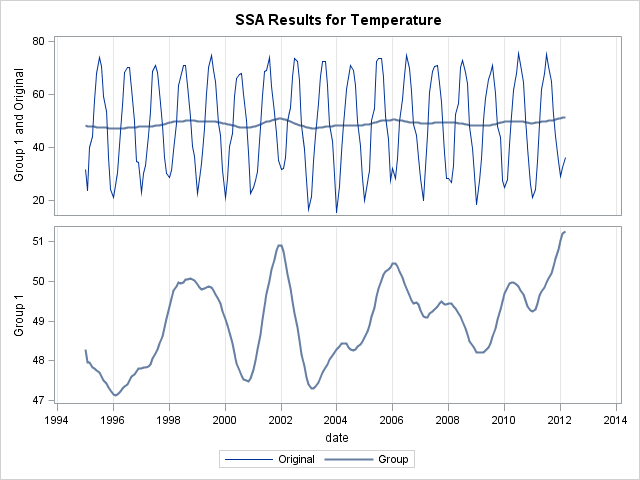Other times series analysis techniques, such as seasonal decomposition, could also be used to analyze these data.

I could have decomposed the original series into more components to identify additional patterns in the data, as is shown in the paper "An Introduction to Singular Spectrum Analysis with SAS/ETS Software". See the documentation for the TIMESERIES procedure for further information about the singular spectrum analysis, how to identify the number of patterns in the data, and its implementation in SAS/ETS software.

In conclusion, my analysis suggests that even after removing seasonal effects of temperature from the data, the long-run average monthly temperature trend is not constant. The first SSA component goes up and down, which shows that additional factors affect the weather in Albany. The most recent Albany winter was one of the warmest since 1995, but the data also show other winters (such as 2002 and 2006) during which Albany experienced higher than average winter temperatures.In yesterday's post, I discussed a "quick and dirty" method to smooth periodic data. However, after I smoothed the data I remarked that the smoother itself was not exactly periodic. At the end points of the periodic interval, the smoother did not have equal slopes and the method does not guarantee that the predicted values are the same. The lack of periodicity occurs because of "edge effects" in the loess algorithm.

In this article I show how to correct these deficiencies to construct a truly periodic smoother. You can download the program used to construct the periodic loess smoother.

### Creating a periodic smoother

The problem, as I discussed in my previous article, is that the loess algorithm behaves differently at the ends of the data range than in the middle. The loess algorithm uses the k observations nearest to x to predict a value at x. In the middle of the data, about k/2 points on either side of x are used to form a prediction at x. However, for observations near the minimum of the data, the algorithm uses about k points to the right of x. For observations near the maximum of the data, the loess algorithm uses about k points to the left of x. This asymmetry leads to the loess curve being aperiodic, even when the data are periodic.

A solution is to translate a copy of the data to the left and to the right before fitting the loess curve. By extending the data, an observation near the minimum of the data still has k/2 points to its right and k/2 points to its left. Furthermore, the points to the right are exactly the k/2 observations with the largest x values.

If you know ahead of time that you only need k/2 points to the left of the original data, you can just translate k/2 points. However, in the following DATA step I translate the entire set of data to the left and to the right. This simplifies the code and is sometimes necessary at the modeling stage of an analysis.

```/* extend data to each side */ data Periodic; set TempData(in=before) TempData TempData(in=after); if before then proportion = proportion - 1; /* (-1,0] */ if after then proportion = proportion + 1; /* (1,2] */ run;```

I want to use 0.167 as the loess smoothing because that was the value used in my previous analysis.. But I need to be careful: I now have three times as much data, so I need to choose a smoothing parameter that is 1/3 smaller. In the following SAS statements, I create a data set to score the predicted values and I call PROC LOESS with the smoothing parameter 0.167 / 3 = 0.0557:

```data Score; do proportion = 0 to 1 by 1/365; output; end;   /* 3 times the data, so use 1/3 the smoothing parameter! */ proc loess data=Periodic plots(only maxpoints=none)=(FitPlot CriterionPlot); model Temperature = Proportion/ smooth=0.0557 interp=cubic; score data=Score; ods output ScoreResults=Fit; run;```

I can now plot the original data and overlay a truly periodic loess curve:

```data Combine; merge TempData Fit(rename=(Proportion=Prop)); run;   proc sgplot data=Combine; scatter x=Proportion y=Temperature / transparency=0.8; scatter x=Prop y=Temp / markerattrs=(color='gray' symbol=CircleFilled) legendlabel="Winter 2011-12"; series x=Prop y=P_Temperature/ lineattrs=(thickness=4px) legendlabel="Periodic smoother"; /* truly periodic */ yaxis grid; title "Temperature in Albany, NY (1995-2012)"; run;```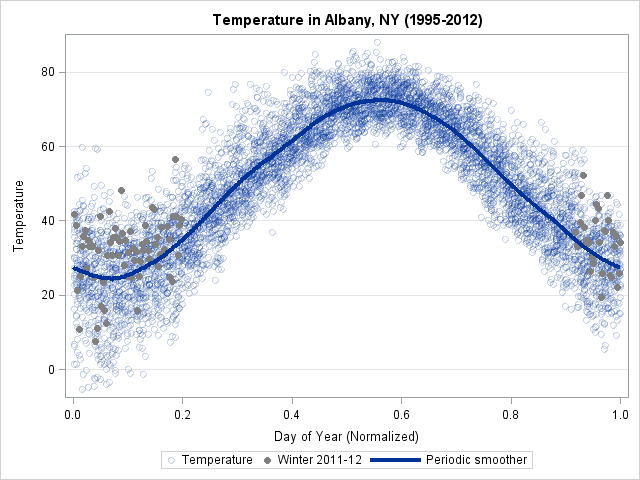Notice that although the predicted values have not changed very much, the slopes of the loess curve at the ends of the data match up. The curve is a periodic smoother for these data. This method (extending the data in both directions) works for smoothers other than the loess smoother. As long as the smoother uses local interpolation, including spline interpolation, this technique should work.

The SAS/IML language has a built-in routine for fitting cubic splines to periodic data. The documentation gives examples of how to use it.Over at the SAS and R blog, Ken Kleinman discussed using polar coordinates to plot time series data for multiple years. The time series plot was reproduced in SAS by my colleague Robert Allison.

The idea of plotting periodic data on a circle is not new. In fact it goes back at least as far as Florence Nightingale who used polar charts to plot the seasonal occurrence of deaths of soldiers during the Crimean War. Her diagrams are today called "Nightingale Roses" or "Coxcombs."

The polar charts created by Kleinman and Allison enable you to see general characteristics of the data and could be useful for comparing the seasonal temperatures of different cities. A city such as Honolulu, Hawaii, that has a small variation in winter-summer temperatures will have a polar diagram for which the data cloud is nearly concentric. Cities with more extreme variation—such as the Albany, NY, data used by Kleinman and Allison—will have a data cloud that is off center. Comparing cities by using polar charts has many of the same advantages and disadvantages as using radar charts.

However, if you want to model the data and display a fitted curve that shows seasonality, then a rectangular coordinate system is better for displaying the data. For me, trying to follow a sinusoidal curve as it winds around a circle requires too much head-tilting!

### Fitting periodic data: The quick-and-dirty way

You can visualize periodic time-series data by "folding" the data onto a scatter plot. The easiest way to do this is to plot the day of the year for each data point. (The day of the year is called the "Julian day" and is easily computed by applying the SAS JULDAYw format.) That produces a scatter plot for which the horizontal axis is in the interval [1, 365], or [1, 366] for leap years. An alternative approach is to transform each date into the interval (0,1] by dividing the Julian day by the number of days in the year (either 365 or 366). The following SAS code performs a "quick and dirty" fit to the temperature data for Albany, NY. The code does the following:

• A DATA step reads the temperatures for Albany, NY, from its Internet URL. This uses the FILENAME statement to access data directly from a URL.
• The Julian day is computed by using the JULDAY3. format.
• The proportion of the year is computed by dividing the Julian day by 365 or 366.
• The winter of 2011-2012 is appended to the data to make it easier to accent those dates while using transparency for the bulk of the data.
• The SGPLOT procedure is used to create a scatter plot of the data and to overlay a loess curve.

```/* Read the data directly from the Internet. This DATA step adapted from Robert Allison's analysis: http://sww.sas.com/~realliso/democd55/albany_ny_circular.sas */ filename webfile url "http://academic.udayton.edu/kissock/http/Weather/gsod95-current/NYALBANY.txt" /* behind a corporate firewall? don't forget the PROXY= option here */ ; data TempData; infile webfile; input month day year Temperature; format date date9.; date=MDY(month, day, year); dayofyear=0; dayofyear=put(date,julday3.); /* incorporate leap years into calculations */ Proportion = dayofyear / put(mdy(12, 31, year), julday3.); label Proportion = "Day of Year (Normalized)"; CurrentWinter = (date >='01dec2011'd & date<='15mar2012'd); if Temperature^=-99 then output; run;   /* Technique to overlay solid markers on transparent markers */ data TempData; set TempData /* full data (transparent markers) */ TempData(where=(CurrentWinter=1) /* special data (solid) */ rename=(Proportion=Prop Temperature=Temp)); run;   proc sgplot data=TempData; scatter x=Proportion y=Temperature / transparency=0.8; scatter x=Prop y=Temp / markerattrs=(color='gray' symbol=CircleFilled) legendlabel="Winter 2011-12"; loess x=Proportion y=Temperature / smooth=0.167 nomarkers lineattrs=(thickness=4px) legendlabel="Loess smoother"; yaxis grid; title "Temperature in Albany, NY (1995-2012)"; run;```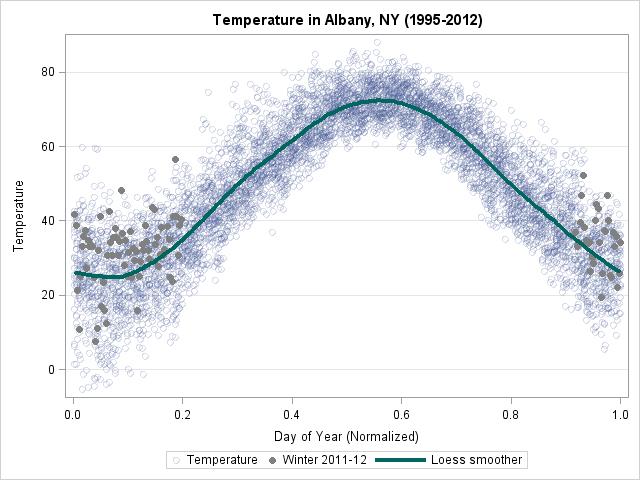A few aspects of the plot are interesting:

• Only about 27% of this past winter's temperatures are below the average temperature, as determined by the loess smoother. This indicates that the Albany winter was warmer than usual—a result that was not apparent in the polar graph.
• The smoother enables you to read the average temperatures for each season.

The observant reader might wonder about the value of the smoothing parameter in the LOESS statement. The smoothing value is 61/365 = 0.167, which was chosen so that the mean temperature of a date in the center of the plot is predicted by using a weighted fit of temperatures for 30 days prior to the date and 30 days after the date. If you ask the LOESS procedure to compute the smoothing value for these data according to the AICC or GCV criterion, both criteria tend to oversmooth these data.

### Creating a periodic smoother

The loess curve for these data is very close to being periodic....but it isn't quite. The value at Proportion=0 and Proportion=1 are almost the same, but the slopes are not. For other periodic data that I've examined, the fitted values have not been equal.

Why does this happen? Because of what are sometimes called "edge effects." The loess algorithm is different near the extremes of the data than it is in the middle. In the middle of that data, about k/2 points on either side of x are used to predict the value at x. However, for observations near Proportion=0, the algorithm uses about k points to the right of x, and for observations near Proportion=1, the loess algorithm uses about k points to the left of x. This asymmetry leads to the loess curve being aperiodic, even when the data are periodic.

Tomorrow I will show how to create a really, truly, honestly periodic smoother for these data.Over at the SAS Discussion Forums, someone asked how to use SAS to fit a Poisson distribution to data. The questioner asked how to fit the distribution but also how to overlay the fitted density on the data and to create a quantile-quantile (Q-Q) plot.

The questioner mentioned that the UNIVARIATE procedure does not fit the Poisson distribution. That is correct: the UNIVARIATE procedure fits continuous distributions, whereas the Poisson distribution is a discrete distribution. Nevertheless, you can fit Poisson data and visualize the results by combining several SAS procedures. This article shows one way to accomplish this. The method also works for other discrete distributions such as the negative binomial and the geometric distribution.

### Do I receive emails at a constant rate?

For data I will use the number of emails that I received one day for each of 19 half-hour periods from 8:00 am to 5:30 pm. If I receive emails at a constant rate during the day, the number of emails in each 30-minute period follows a Poisson distribution. The following DATA step defines the data; PROC FREQ tabulates and plots the sample distribution:

```/* number of emails received in each half-hour period 8:00am - 5:30pm on a weekday. */ data MyData; input N @@; /* 8a 9a 10a 11a 12p 1p 2p 3p 4p 5p */ datalines; 7 7 13 9 8 8 9 9 5 6 6 9 5 10 4 5 3 8 4 ; run; /* Tabulate counts and plot data */ proc freq data=MyData; tables N / out=FreqOut plots=FreqPlot(scale=percent); run;```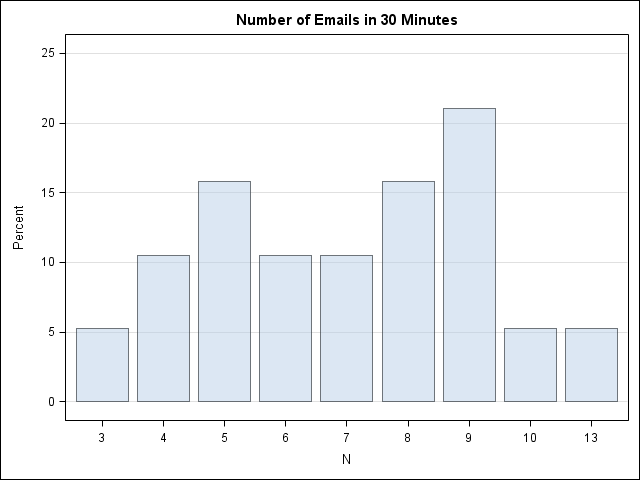The mean of the data is about 7. A Poisson(7) distribution looks approximately normal—which these data do not. On the other hand, there are less than 20 observations in the data, so let's proceed with the fit. (I actually looked at several days of email before I found a day that I could model as Poisson, so these data are NOT a random sample!)

### Fit the data

The first step is to fit the Poisson parameter to the data. You can do this in PROC GENMOD by by using the DIST= option to specify a Poisson distribution. Notice that I do not specify any explanatory variables, which means that I am fitting the mean of the data.

```/* 1. Estimate the rate parameter with PROC GENMOD: http://support.sas.com/kb/24/166.html */ proc genmod data=MyData; model N = / dist=poisson; output out=PoissonFit p=lambda; run;```

At this point you should look at the goodness-of-fit and parameter estimates tables that PROC GENMOD creates to see how well the model fits the data. I will skip these steps.

### Compute the fitted density

The P= option on the OUTPUT statement outputs the mean, which is also the parameter estimate for the fitted Poisson distribution. The mean is about 7.1. The following statements set a macro variable to that value and create a data set (PMF) that contains the Poisson(7.1) density for various x values. In a subsequent step, I'll overlay this fitted density on the empirical density.

```/* 2. Compute Poisson density for estimated parameter value */ /* 2.1 Create macro variable with parameter estimate */ data _null_; set PoissonFit; call symputx("Lambda", Lambda); stop; run;   /* 2.2 Use PDF function for range of x values */ data PMF; do t = 0 to 13; /* 0 to max(x) */ Y = pdf("Poisson", t, &Lambda); output; end; run;```

### Overlay the empirical and fitted densities

I want to overlay the discrete density on a bar chart of the data. One way to visualize the discrete density is as a scatter plot of (x, pdf(x)) values that represent the fitted density at x=0, 1,...,13. Unfortunately, you cannot use the VBAR and the SCATTER statements in the same SGPLOT call to overlay a bar chart and a scatter plot. However, in SAS 9.3 you can use the VBARPARM statement together with the SCATTER statement. (Thanks to "PGStats" for this suggestion.) The VBARPARM statement requires that you compute the heights of the bars yourself, but the heights are easily constructed from the PROC FREQ output that was created earlier:

```/* 3. Use bar chart to plot data. To overlay a bar chart and scatter plot, use the VBARPARM stmt instead of VBAR. */ data Discrete; merge FreqOut PMF; Prop = Percent / 100; /* convert to same scale as PDF */ run;   /* 3.2 Overlay VBARPARM and scatter plot of (x, pdf(x)) */ proc sgplot data=Discrete; /* VBARPARM is SAS 9.3 stmt */ vbarparm category=N response=Prop / legendlabel='Sample'; scatter x=T y=Y / legendlabel='PMF' markerattrs=GraphDataDefault(symbol=CIRCLEFILLED size=10); title "Emails per 30-Minute Period and Poisson Distribution"; run;```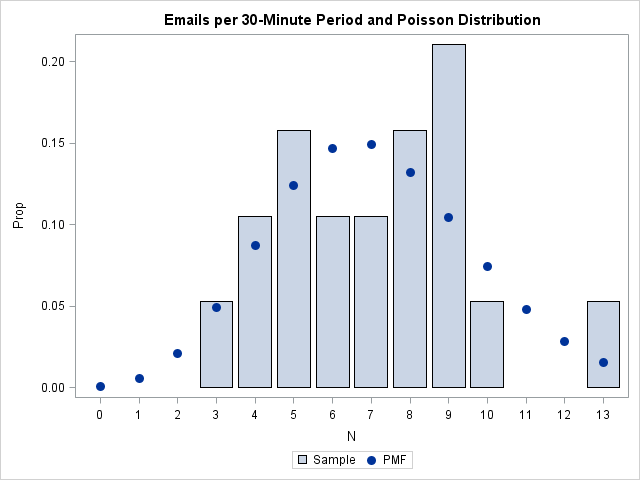### Create a discrete Q-Q plot

On the Discussion Forum, the questioner asked for a quantile-quantile plot. I don't know whether I've ever seen a Q-Q plot for a discrete distribution before; usually they are shown for continuous distributions. However, you can create a discrete Q-Q plot by following exactly the same steps that I described in my previous article on how to compute a Q-Q plot:

```/* 4. Create a Q-Q plot */ /* 4.1 Compute theoretical quantiles */ proc sort data=MyData; by N; run; /* 1 */ data QQ; set MyData nobs=nobs; v = (_N_ - 0.375) / (nobs + 0.25); /* 2 */ q = quantile("Poisson", v, &Lambda); /* 3 */ run;   proc sgplot data=QQ noautolegend; /* 4 */ scatter x=q y=N; lineparm x=0 y=0 slope=1; /* SAS 9.3 statement */ xaxis label="Poisson Quantiles" grid; yaxis label="Observed Data" grid; title "Poisson Q-Q Plot of Emails"; run;```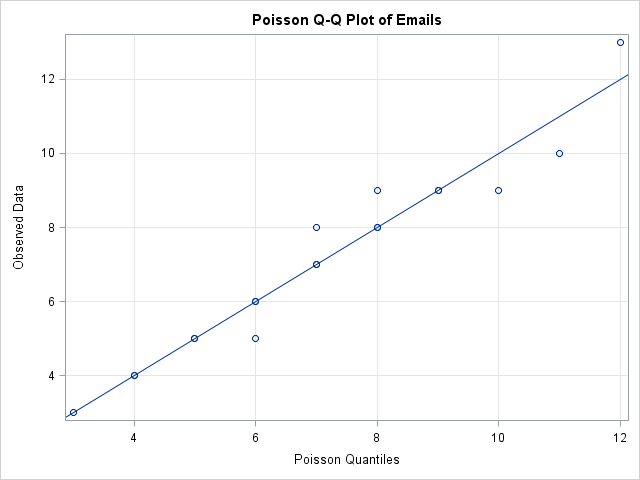I've created a discrete Q-Q plot, but is it useful? A drawback appears to be that the discrete Q-Q plot suffers from overplotting, whereas a continuous Q-Q plot does not. A continuous CDF function is one-to-one so the quantiles of the ranks of the data are unique. In contrast, the CDF function for a discrete distribution is a step function, which leads to duplicated quantiles and overplotting.

For example, in the discrete Poisson Q-Q plot for my email, there are 19 observations, but only 13 points are visible in the Q-Q plot due to overplotting. If I analyze 10 days of my email traffic, I could get 190 observations, but the Q-Q plot might show only a fraction of those points. (In simulated data, there were only 25 unique values in 190 observations drawn from a Poisson(7) distribution.)

The fact that I don't often see discrete Q-Q plots bothered me, so I did a little research. I found a reference to discrete Q-Q plots on p. 126 of Computational Statistics Handbook with MATLAB where it says:

Quantile plots...are primarily used for continuous data. We would like to have a similar technique for graphically comparing the shapes of discrete distributions. Hoaglin and Tukey  developed several plots to accomplish this [including] the Poissonness plot.

That sounds interesting! A future blog post will present an alternative way to visualize the fit of a Poisson model.